# 2.6: Equilibrium

What you’ll learn to do: explain market equilibrium, surplus, and shortage

In this section, you’ll learn how supply and demand interact to determine the price in a market.

learning objectives

• Explain equilibrium price and quantity
• Explain equilibrium price and quantity

## Surpluses and Shortages

In order to understand market equilibrium, we need to start with the laws of demand and supply. Recall that the law of demand says that as price decreases, consumers demand a higher quantity. Similarly, the law of supply says that when price decreases, producers supply a lower quantity.

Because the graphs for demand and supply curves both have price on the vertical axis and quantity on the horizontal axis, the demand curve and supply curve for a particular good or service can appear on the same graph. Together, demand and supply determine the price and the quantity that will be bought and sold in a market. These relationships are shown as the demand and supply curves in Figure $$\PageIndex{1}$$, which is based on the data in Table $$\PageIndex{1}$$, below.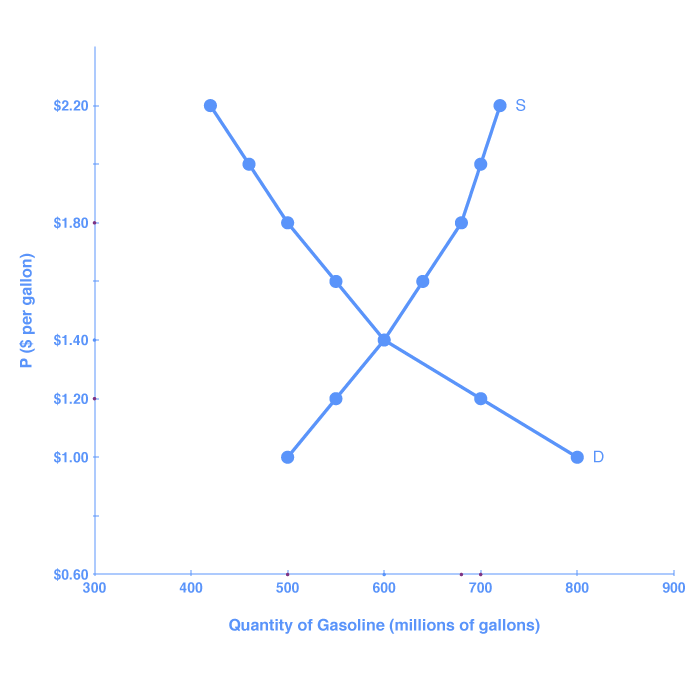Figure $$\PageIndex{1}$$. The supply and demand curves for gasoline.

Table $$\PageIndex{1}$$. Price, Quantity Demanded, and Quantity Supplied
Price (per gallon) Quantity demanded (millions of gallons) Quantity supplied (millions of gallons)
$1.00 800 500$1.20 700 550
$1.40 600 600$1.60 550 640
$1.80 500 680$2.00 460 700
$2.20 420 720 If you look at either Figure $$\PageIndex{1}$$ or Table $$\PageIndex{1}$$, you’ll see that at most prices the amount that consumers want to buy (which we call the quantity demanded) is different from the amount that producers want to sell (which we call the quantity supplied). What does it mean when the quantity demanded and the quantity supplied aren’t the same? The answer is: a surplus or a shortage. Surplus or Excess Supply Let’s consider one scenario in which the amount that producers want to sell doesn’t match the amount that consumers want to buy. Consider our gasoline market example. Imagine that the price of a gallon of gasoline were$1.80 per gallon. This price is illustrated by the dashed horizontal line at the price of $1.80 per gallon in Figure $$\PageIndex{2}$$, below.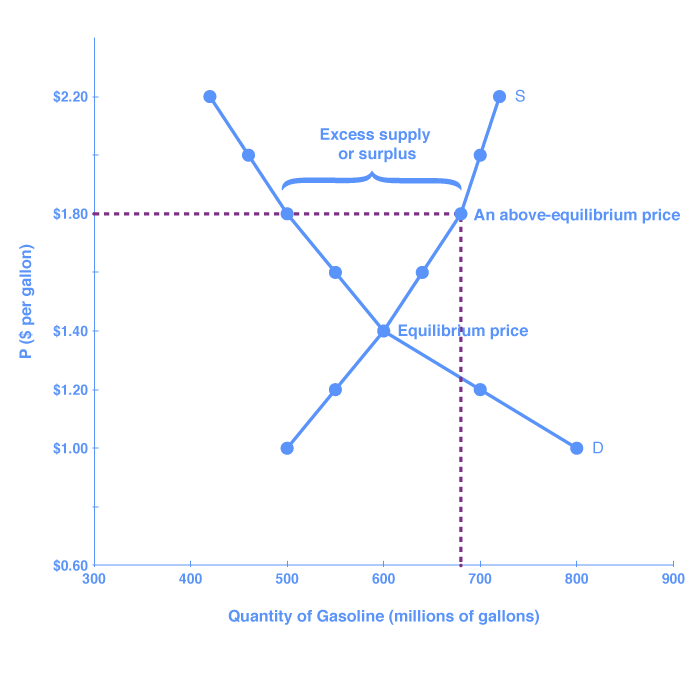Figure $$\PageIndex{2}$$. A price above equilibrium creates a surplus. At this price, the quantity demanded is 500 gallons, and the quantity of gasoline supplied is 680 gallons. You can also find these numbers in Table $$\PageIndex{1}$$, above. Now, compare the quantity demanded and quantity supplied at this price. Quantity supplied (680) is greater than quantity demanded (500). Or, to put it in words, the amount that producers want to sell is greater than the amount that consumers want to buy. We call this a situation of excess supply (since Qs > Qd) or a surplus. Note that whenever we compare supply and demand, it’s in the context of a specific price—in this case,$1.80 per gallon.

With a surplus, gasoline accumulates at gas stations, in tanker trucks, in pipelines, and at oil refineries. This accumulation puts pressure on gasoline sellers. If a surplus remains unsold, those firms involved in making and selling gasoline are not receiving enough cash to pay their workers and cover their expenses. In this situation, some firms will want to cut prices, because it is better to sell at a lower price than not to sell at all. Once some sellers start cutting prices; others will follow to avoid losing sales. These price reductions will, in turn, stimulate a higher quantity demanded.

How far will the price fall? Whenever there is a surplus, the price will drop until the surplus goes away. When the surplus is eliminated, the quantity supplied just equals the quantity demanded—that is, the amount that producers want to sell exactly equals the amount that consumers want to buy. We call this equilibrium, which means “balance.” In this case, the equilibrium occurs at a price of $1.40 per gallon and at a quantity of 600 gallons. You can see this in Figure $$\PageIndex{2}$$ (and Figure $$\PageIndex{1}$$) where the supply and demand curves cross. You can also find it in Table $$\PageIndex{1}$$ (the numbers in bold). practice question $$\PageIndex{1}$$ The market for cement in a small town is defined by a demand curve that passes through the points ($50/ton, 300 tons), ($100/ton, 200 tons), ($150/ton, 100 tons). Meanwhile we know the supply curve passes through the points ($150/ton, 300 tons), ($100/ton, 200 tons), ($50/ton, 100 tons). If the market starts in a surplus of 200 tons, how much must the price change before the market is in equilibrium? 1. decrease by$50/ton
2. increase by $50/ton 3. decrease by$100/ton

A. If there is a surplus of 200 tons then the price must be $150 (300 tons supplied - 100 tons demanded = 200 ton surplus). Equilibrium occurs at$100/ton, so the price must decrease by $50. practice question $$\PageIndex{2}$$ Consider the combined yearly onion supply and demand schedules for one local supermarket below. Currently the market is at the equilibrium price A. Fill in the values for A and B that would cause market equilibrium to occur at that price. Price (per Onion) Quantity demanded (thousands of onions) Quantity supplied (thousands of onions)$0.50 100 10
$0.70 90 D? A? B? 80$1.10 70 120
$1.30 C? 160$1.50 50 200
$1.70 40 220 1. A:$0.90; B: 90
2. A: $1.50; B: 80 3. A:$0.90; B: 80

C. To find the free market equilibrium set the quantity demanded B equal to the quantity supplied (80). The equilibrium price A must be in between $0.70 and$1.10 (i.e. $0.90) Shortage or Excess Demand Let’s return to our gasoline problem. Suppose that the price is$1.20 per gallon, as the dashed horizontal line at this price in Figure 3, below, shows. At this price, the quantity demanded is 700 gallons, and the quantity supplied is 550 gallons.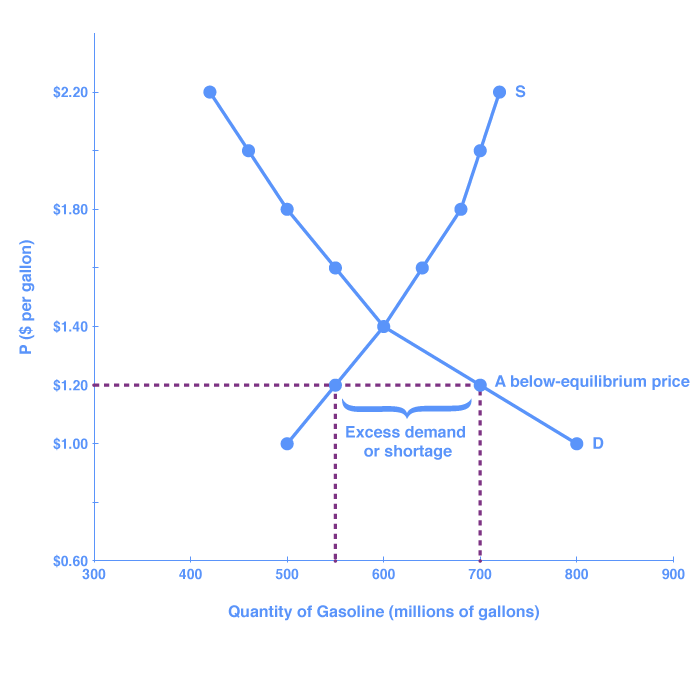Figure $$\PageIndex{3}$$. A price below equilibrium creates a shortage.

Quantity supplied (550) is less than quantity demanded (700). Or, to put it in words, the amount that producers want to sell is less than the amount that consumers want to buy. We call this a situation of excess demand (since Qd > Qs) or a shortage.

In this situation, eager gasoline buyers mob the gas stations, only to find many stations running short of fuel. Oil companies and gas stations recognize that they have an opportunity to make higher profits by selling what gasoline they have at a higher price. These price increases will stimulate the quantity supplied and reduce the quantity demanded. As this occurs, the shortage will decrease. How far will the price rise? The price will rise until the shortage is eliminated and the quantity supplied equals quantity demanded. In other words, the market will be in equilibrium again. As before, the equilibrium occurs at a price of $1.40 per gallon and at a quantity of 600 gallons. Generally any time the price for a good is below the equilibrium level, incentives built into the structure of demand and supply will create pressures for the price to rise. Similarly, any time the price for a good is above the equilibrium level, similar pressures will generally cause the price to fall. As you can see, the quantity supplied or quantity demanded in a free market will correct over time to restore balance, or equilibrium. ## Equilibrium, Price, and Quantity Equilibrium: Where Supply and Demand Intersect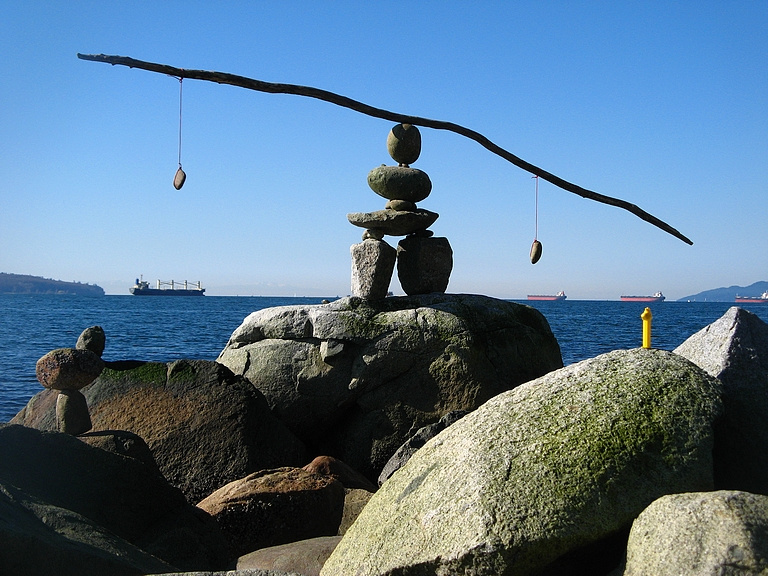Figure $$\PageIndex{4}$$. Equilibrium is the point where the amount that buyers want to buy matches the point where sellers want to sell. When two lines on a diagram cross, this intersection usually means something. On a graph, the point where the supply curve (S) and the demand curve (D) intersect is the equilibrium. The equilibrium price is the only price where the desires of consumers and the desires of producers agree—that is, where the amount of the product that consumers want to buy (quantity demanded) is equal to the amount producers want to sell (quantity supplied). This mutually desired amount is called the equilibrium quantity. At any other price, the quantity demanded does not equal the quantity supplied, so the market is not in equilibrium at that price. It should be clear from the previous discussions of surpluses and shortages, that if a market is not in equilibrium, market forces will push the market to the equilibrium. If you have only the demand and supply schedules, and no graph, you can find the equilibrium by looking for the price level on the tables where the quantity demanded and the quantity supplied are equal (again, the numbers in bold in Table $$\PageIndex{1}$$ indicate this point). Finding equilibrium with algebra We’ve just explained two ways of finding a market equilibrium: by looking at a table showing the quantity demanded and supplied at different prices, and by looking at a graph of demand and supply. We can also identify the equilibrium with a little algebra if we have equations for the supply and demand curves. Let’s practice solving a few equations that you will see later in the course. Right now, we are only going to focus on the math. Later you’ll learn why these models work the way they do, but let’s start by focusing on solving the equations. Suppose that the demand for soda is given by the following equation: Qd = 16 – 2P , where Qd is the amount of soda that consumers want to buy (i.e., quantity demanded), and P is the price of soda. Suppose the supply of soda is Qs = 2 + 5P , where Qs is the amount of soda that producers will supply (i.e., quantity supplied). (Remember, these are simple equations for lines). Finally, recall that the soda market converges to the point where supply equals demand, or Qd = Qs , We now have a system of three equations and three unknowns (Qd, Qs, and P), which we can solve with algebra. Since $Qd$$= Qs$ , we can set the demand and supply equations equal to each other: Qd = Qs 16 − 2P = 2 + 5P Step 1: Isolate the variable by adding 2P to both sides of the equation, and subtracting 2 from both sides. 16 − 2P = 2 + 5P −2 + 2P = −2 + 2P 14 = 7P Step 2: Simplify the equation by dividing both sides by 7. $\begin{array}{c}\frac{14}{7}\end{array}$$\begin{array}{c}=\\ \frac{7P}{7}\end{array}$ 2 = P The equilibrium price of soda, that is, the price where Qs = Qd will be$2. Now we want to determine the quantity amount of soda. We can do this by plugging the equilibrium price into either the equation showing the demand for soda or the equation showing the supply of soda. Let’s use demand. Remember, the formula for quantity demanded is the following:

Qd = 16 − 2P

Taking the price of $2, and plugging it into the demand equation, we get Qd = 16 – 2(2) Qd = 16 – 4 Qd = 12 So, if the price is$2 each, consumers will purchase 12. How much will producers supply, or what is the quantity supplied? Taking the price of $2, and plugging it into the equation for quantity supplied, we get the following: Qs = 2 + 5P Qs = 2 + 5(2) Qs = 2 + 10 Qs = 12 Now, if the price is$2 each, producers will supply 12 sodas. This means that we did our math correctly, since

Qd = Q$s$

and both Qd and Qs are equal to 12. That confirms that we’ve found the equilibrium quantity.

Watch this video for a closer look at market equilibrium:

Equilibrium occurs at the point where quantity supplied = quantity demanded.

Equilibrium and Economic Efficiency

Equilibrium is important to create both a balanced market and an efficient market. If a market is at its equilibrium price and quantity, then it has no reason to move away from that point, because it’s balancing the quantity supplied and the quantity demanded. However, if a market is not at equilibrium, then economic pressures arise to move the market toward the equilibrium price and equilibrium quantity. This happens either because there is more supply than what the market is demanding or because there is more demand than the market is supplying. This balance is a natural function of a free-market economy.

Also, a competitive market that is operating at equilibrium is an efficient market. Economists typically define efficiency in this way: when it is impossible to improve the situation of one party without imposing a cost on another. Conversely, if a situation is inefficient, it becomes possible to benefit at least one party without imposing costs on others.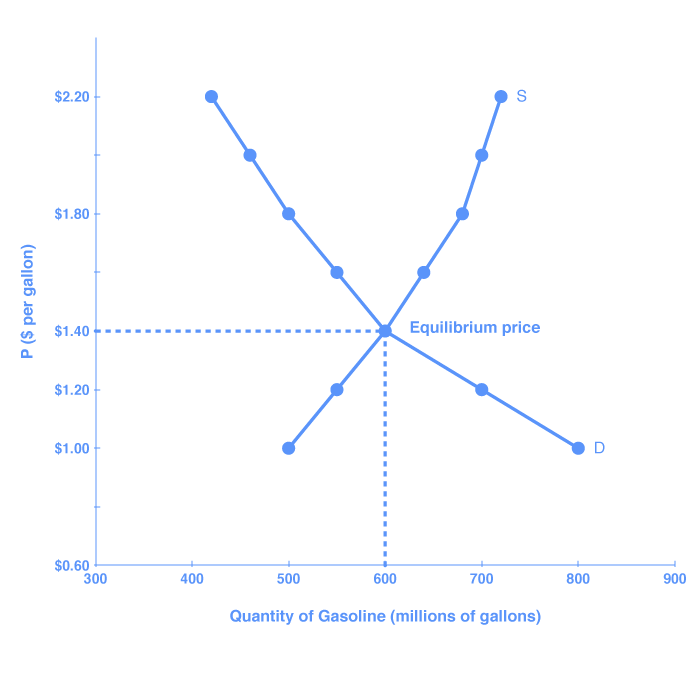Figure $$\PageIndex{5}$$. Demand and Supply for Gasoline: Equilibrium. At this equilibrium point, the market is efficient because the optimal amount of gasoline is being produced and consumed.

Efficiency in the demand and supply model has the same basic meaning: the economy is getting as much benefit as possible from its scarce resources, and all the possible gains from trade have been achieved. In other words, the optimal amount of each good and service is being produced and consumed.

practice Quesion $$\PageIndex{3}$$

The market for corn is defined by the graph below. If the price is set at $20/ton will this result in a surplus or shortage? What is the magnitude of this surplus or shortage?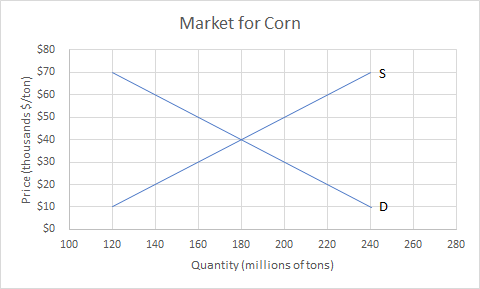1. shortage of 120 million tons 2. surplus of 80 million tons 3. shortage of 80 million tons Answer C - At$20/ton the quantity supplied is 140 million tons, while the quantity demanded is 220 million tons. Since the quantity demanded is greater than the quantity supplied at the given price, then there is a shortage of 220-140=80 million tons

practice question $$\PageIndex{4}$$

As member of the “Hungry for Apples?” national advertising campaign you are charged with analyzing future trends in Apple prices. The estimated demand and supply curve for apples is displayed below. If the current price is $100/ton, by how much must prices change before the market is in equilibrium? Is there currently a surplus or shortage in the current market for apples?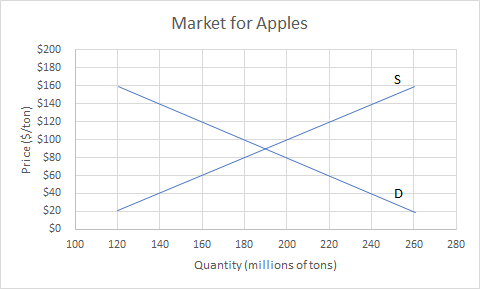1. There is a shortage. The price must increase by$10/ton.
2. There is a shortage. The price must drop by $10/ton. 3. There is a surplus. The price must drop by$10/ton

C - The equilibrium price displayed above occurs at $90/ton. If the current price is$100/ton then there must be a surplus. Therefore the price must drop by $10/ton to arrive at equilibrium. Finding Equilibrium using the Four-Step Process We know that equilibrium is the place where the supply and demand curves intersect, or the point where buyers want to buy the same amount that sellers want to sell. Let’s take a closer look at how to find the equilibrium point using the four-step process. These steps explain how to first, draw the demand a supply curves on a graph and find the equilibrium. Next, consider how an economic change (e.g. a natural disaster, a change in production technology, a change in tastes and preferences, income, etc.) might affect supply or demand, then make adjustments to the graph to identify the new equilibrium point. Step 1. Draw demand and supply curves showing the market before the economic change took place. Think about the shift variables for demand, and the shift variables for supply. Using this diagram, find the initial equilibrium values for price and quantity. Step 2. Decide whether the economic change being analyzed affects demand or supply. In other words, does the event refer to something in the list of demand shift variables or supply shift variables? Step 3. Determine whether the effect on demand or supply causes the curve to shift to the right or to the left, and sketch the new demand or supply curve on the diagram. In other words, does the event increase or decrease the amount consumers want to buy or the amount producers want to sell? Step 4. Identify the new equilibrium, and then compare the original equilibrium price and quantity to the new equilibrium price and quantity. Let’s consider one example that involves a shift in supply and one that involves a shift in demand. Then we will consider an example where both supply and demand shift. Exercise $$\PageIndex{1}$$: Good weather for salmon fishing Let’s suppose that during the summer of 2015, weather conditions were excellent for commercial salmon fishing off the California coast. Heavy rains meant higher than normal levels of water in the rivers, which helps the salmon to breed. Slightly cooler ocean temperatures stimulated the growth of plankton, the microscopic organisms at the bottom of the ocean food chain, providing everything in the ocean with a hearty food supply. The ocean stayed calm during fishing season, so commercial fishing operations did not lose many days to bad weather. How did these climate conditions affect the quantity and price of salmon? Let’s consider this situation using the four-step process and the data below. Table 1. Salmon Fishing Price per Pound Quantity Supplied in 2014 Quantity Supplied in 2015 Quantity Demanded$2.00 80 400 840
$2.25 120 480 680$2.50 160 550 550
$2.75 200 600 450$3.00 230 640 350
$3.25 250 670 250$3.50 270 700 200

Let’s walk through the four steps together using this example, and see how the graph changes. Use the interactive graph below (Figure $$\PageIndex{6}$$) by clicking on the arrows at the bottom of the activity to navigate through the steps.

Figure $$\PageIndex{6}$$ (Interactive Graph). Good Weather for Salmon Fishing: The Four-Step Process.

In short, good weather conditions increased supply of the California commercial salmon. The result was a higher equilibrium quantity of salmon bought and sold in the market at a lower price.

Exercise $$\PageIndex{2}$$: newspapers and the internet

According to the Pew Research Center for People and the Press, more and more people, especially younger people, are getting their news from online and digital sources. The majority of U.S. adults now own smartphones or tablets, and most of those Americans say they use them in part to get the news. From 2004 to 2012, the share of Americans who reported getting their news from digital sources increased from 24 percent to 39 percent. How has this trend affected consumption of print news media and radio and television news? Figure $$\PageIndex{7}$$ and the text below illustrate the four-step analysis used to answer this question.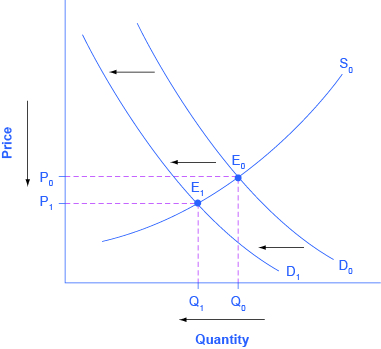Figure $$\PageIndex{7}$$ Graph depicting the changing market for print news.

Step 1. Draw a demand and supply model to think about what the market looked like before the event.

The demand curve D0 and the supply curve S0 show the original relationships. In this case, the curves are drawn without specific numbers on the price and quantity axis.

Step 2. Did the change described affect supply or demand?

A change in tastes, from traditional news sources (print, radio, and television) to digital sources, caused a change in demand for the former

Step 3. Was the effect on demand positive or negative?

A shift to digital news sources will tend to mean a lower quantity demanded of traditional news sources at every given price, causing the demand curve for print and other traditional news sources to shift to the left, from D0 to D1.

Step 4. Compare the new equilibrium price and quantity to the original equilibrium price.

The new equilibrium (E1) occurs at a lower quantity and a lower price than the original equilibrium (E0).

The decline in print news reading predates 2004. Print newspaper circulation peaked in 1973 and has declined since then due to competition from television and radio news. In 1991, 55 percent of Americans indicated that they got their news from print sources, while only 29 percent did so in 2012. Radio news has followed a similar path in recent decades, with the share of Americans getting their news from radio declining from 54 percent in 1991 to 33 percent in 2012. Television news has held its own during the last fifteen years, with the market share staying in the mid to upper fifties. What does this suggest for the future, given that two-thirds of Americans under thirty years old say they don’t get their news from television at all?

practice question $$\PageIndex{5}$$

Note: Using a pen and paper to illustrate supply and demand shifts could be helpful for this question

A recent health study reveals that red wine may be linked to increased longevity. At the same time, a severe drought is affecting wine production is Southern California. How will the new equilibrium quantity and price compare with the original equilibrium before the health study and drought?

1. The equilibrium price and quantity in the wine market will increase relative to the old equilibrium.
2. The equilibrium price in the wine market will increase relative the old equilibrium, yet the effect on quantity is impossible to determine.
3. The equilibrium quantity in the wine market will increase relative the old equilibrium, yet the effect on price is impossible to determine

b - A severe drought will shift the supply curve for wine to the left, while the health study will shift the demand curve right. This will result in higher equilibrium prices yet the effect on quantity is ambiguous, and depends on the magnitude of the supply and demand shifts.

practice question $$\PageIndex{6}$$

In the past year the price of pork, the main ingredient in hot dogs, has decreased by 20%. At the same time the price of hot dog buns has also dropped 20%. How will the new equilibrium quantity and price for hot dogs compare with the original equilibrium before these price changes?

Hint: Using a pen and paper to illustrate supply and demand shifts could be helpful for this question

1. The equilibrium quantity in the hot dog market will increase relative the old equilibrium, yet the effect on price is impossible to determine.
2. The equilibrium price in the hot dog market will increase relative the old equilibrium, yet the effect on quantity is impossible to determine.
3. The equilibrium price and quantity in the hot dog market will increase relative the old equilibrium.

A. Lower cost of hot dog input pork will shift the supply curve to the right, while a drop in the price of hot dog buns, a complement, shifts the demand curve right. The resulting equilibrium quantity will have increased, while the effect on equilibrium price is ambiguous, and depends on the magnitude of supply and demand shifts.

Try It

Play the simulation below multiple times to see how different choices lead to different outcomes. All simulations allow unlimited attempts so that you can gain experience applying the concepts.

## Practice: Equilibrium

You have seen how changes in weather can influence supply and changes in consumer preferences can reduce demand, but what happens when both supply and demand are changing? Often changes in an economy affect both the supply and the demand curves, making it more difficult to assess the impact on the equilibrium price. Let’s review one such example.Figure $$\PageIndex{8}$$. Money and Mail. How do changes at the post office impact other aspects of the economy?

First, consider the following questions:

1. Suppose postal workers are successful in obtaining a pay raise from the U.S. Postal Service. Will this affect the supply or the demand for first-class mail? Why? Which determinant of demand or supply is being affected? Show graphically with before and after curves on the same axes. How will this change the equilibrium price and quantity of first-class mail?
2. How do you imagine the invention of email and text messaging affected the market for first-class mail? Why? Which determinant of demand or supply is being affected? Show graphically with before and after curves on the same axes. How will this change the equilibrium price and quantity of first-class mail?
3. Suppose that postal workers get a pay raise and email and text messaging become common. What will the combined impact be on the equilibrium price and quantity of first-class mail?

In order to complete a complex analysis like this it’s helpful to tackle the parts separately and then combine them, while thinking about possible interactions between the two parts that might affect the overall outcome. Let’s use the four-step process.

Exercise $$\PageIndex{3}$$: Postal service

Part 1: A Pay Raise for Postal Workers

Step 1. Draw a demand and supply model to illustrate what the market for the U.S. Postal Service looks like before this scenario starts. The demand curve D and the supply curve S show the original relationships.Figure $$\PageIndex{9}$$. The supply and demand curves for the U.S. Postal Service after a pay raise for postal workers.

Step 2. Will a pay raise for postal workers affect supply or demand?

Labor compensation is a cost of production. A change in production costs cause a change in supply for the Postal Service.

Step 3. Is the effect on supply positive or negative?

Higher labor compensation leads to a lower quantity supplied of postal services at every given price, causing the supply curve for postal services to shift to the left, from S to S1.

Step 4. Compare the new equilibrium price and quantity to the original equilibrium price.

The new equilibrium occurs at a lower quantity and a higher price than the original equilibrium.

A pay raise for postal workers would represent an increase in the cost of production for the Postal Service. Production costs are a factor that influences supply; thus, the pay raise should decrease the supply of first-class mail, shifting the supply curve vertically by the amount of the pay raise. Intuitively, all else held constant, the Postal Service would like to charge a higher price that incorporates the higher cost of production. That is not to say the higher price will stick. From the graph (Figure $$\PageIndex{9}$$), it should be clear that at that higher price, the quantity supplied is greater than the quantity demanded—thus there would be a surplus, indicating that the price the Postal Service desires is not an equilibrium price. Or to put it differently, at the original price (P1), the decrease in supply causes a shortage driving up the price to a new equilibrium level (P2). Note that the price doesn’t rise by the full amount of the pay increase. In short, a leftward shift in the supply curve causes a movement up the demand curve, resulting in a lower equilibrium quantity (Q2) and a higher equilibrium price (P2).

Part 2: The Effect of Email and Text Messaging

Step 1. We’ve already seen how a pay raise will shift the supply curve to the left. Now let’s consider how the invention of email and text messaging affects the market for first-class mail. Begin by drawing a demand and supply model reflecting this relationship.Figure $$\PageIndex{10}$$. The impact of email and text messages on the snail mail market.

Step 2. Does email and text messaging affect supply or demand?

A change in tastes away from snail mail toward digital messages will cause a change in demand for the Postal Service.

Step 3. Is the effect on demand positive or negative?

A change in tastes away from snail mail toward digital messages causes lower quantity demanded of postal services at every given price, causing the demand curve for postal services to shift to the left, from D to D1.

Step 4. Compare the new equilibrium price and quantity to the original equilibrium price.

The new equilibrium occurs at a lower quantity and a lower price than the original equilibrium.

To summarize, since many people find email and texting more convenient than sending a letter, we can assume that tastes and preferences for first-class mail will decline. This decrease in demand is shown by a leftward shift in the demand curve and a movement along the supply curve, which creates a surplus in first-class mail at the original price (shown as P2). The shortage causes a decrease in the equilibrium price (to P3) and a decrease in the equilibrium quantity (to Q3). Intuitively, less demand for first-class mail leads to a lower equilibrium quantity and (ceteris paribus) a lower equilibrium price.

Part 3: Combining Factors

Parts 1 and 2 are straightforward, but when we put them together it becomes more complex. Think about it this way: in Part 1, the equilibrium quantity fell due to decreased supply. In Part 2, the equilibrium quantity also fell, this time due to the decreased demand. So putting the two parts together, we would expect to see the final equilibrium quantity (Q3) to be smaller than the original equilibrium quantity (Q1). So far, so good.

Now consider what happens to the price. In Part 1, the equilibrium price increased due to the reduction in supply. But in Part 2, the equilibrium price decreased due to the decrease in demand! What will happen to the equilibrium price? The net effect on price can’t be determined without knowing which curve shifts more, demand or supply. The equilibrium price could increase, decrease, or stay the same. You just can’t tell from graphical analysis alone. This is not unusual. When both curves shift, typically we can determine the overall effect on price or on quantity, but not on both. In this case, we determined the overall effect on the equilibrium quantity, but not on the equilibrium price.Figure $$\PageIndex{11}$$. Decreased supply and decreased demand cause the equilibrium quantity to fall (Q3 is be smaller than the original equilibrium quantity of Q1). It is hard to pinpoint what happens to the price, however, as it may decline with the shrinking demand, but also increase with production costs.

practice Question $$\PageIndex{7}$$

The past year has had very favorable growing conditions for oranges in California. At the same time, a new study reports that eating citrus fruits are linked to better health and longevity. As an economist, what movements in equilibrium quantity and price do you predict given the above information?

1. Equilibrium quantity and price will increase.
2. Equilibrium quantity will increase while price cannot be determined.
3. Equilibrium price will increase while quantity cannot be determined.

B. While rightward shifts of supply and demand will lead to a greater equilibrium quantity, it is impossible to determine the new equilibrium price without knowing which curve shifts more. You cannot tell from graphical analysis alone.

practice Question $$\PageIndex{8}$$

Due to the negative health effects of smoking the number of cigarette consumers has steadily decreased over the past decade. During the same time period more permissive immigration laws has allowed for cheaper tobacco harvesting using migrant labor. As an economist, what movements in equilibrium quantity and price do you predict given the above information?

1. Equilibrium quantity will decrease while price cannot be determined.
2. Equilibrium price will decrease while quantity cannot be determined.

C. Lower production costs will shift the supply curve rightwards, while fewer consumers will move demand leftwards. This results in a lower equilibrium price, yet quantity cannot be determined without knowing which curve shifts more.

practice Questions $$\PageIndex{9}$$

Here are the equations for the demand and supply curves:

Demand curve: Qd = 3300− 2 P

Supply curve: Qs = 500 + 8P

Compute the equilibrium price value.

280

Compute the equilibrium quantity value:

2740

Suppose Congress cuts personal income tax rates. How would this affect the market for air conditioners?

1. Supply curve shifts right.
2. Supply curve shifts left.
3. Demand curve shifts right.
4. Demand curve shifts left.

c. Demand Curve shifts right

Why does the demand or supply curve shift the way it does and what element of demand caused the shift? How does that affect the equilibrium price and quantity?

A decrease in tax rates effectively increases your income. An increase in income generally increases demand. Therefore demand curve shifts to the right to show an increase in the equilibrium price and quantity.

Suppose that air conditioner workers accept a pay cut of 2 dollars per hour. How would this affect the market for air conditioners?

1. Supply curve shifts right.
2. Supply curve shifts left.
3. Demand curve shifts right.
4. Demand curve shifts left.